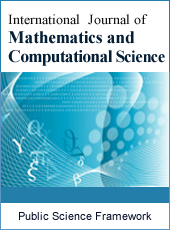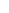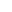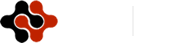International Journal of Mathematics and Computational Science
Articles Information
International Journal of Mathematics and Computational Science, Vol.4, No.2, Jun. 2018, Pub. Date: Jun. 7, 2018
Total Variation Distance Between Poisson Distribution and Polya Distribution and It’s Non-uniform Upper Bound
 Bright Okore Osu, Department of Mathematics, Michael Okpara University of Agriculture, Umudike, Nigeria.
 Samson Ogu–Egege, Department of Mathematics, Abia State University, Uturu, Nigeria.
 Emmanuel Inyang, Department of Mathematics, Abia State University, Uturu, Nigeria.
The approximation of distribution due to Egege et al  is further extended to a wider approximation called total variation distances between Poisson and Pόlya distribution, using Stein’s Chen method and ω -function to give a non-uniform bound. In this work it was found that the results obtain for non-uniform are better than the results obtained for uniform bound in literature. Polya distribution approximate Poisson sufficiently enough provided r is close to n and N is large. If the upper bound is very small then a good approximation is obtained.
The approximation of distribution due to Egege et al  is further extended to a wider approximation called total variation distances between Poisson and Pόlya distribution, using Stein’s Chen method and ω -function to give a non-uniform bound. In this work it was found that the results obtain for non-uniform are better than the results obtained for uniform bound in literature. Polya distribution approximate Poisson sufficiently enough provided r is close to n and N
 Samson O Egege, Bright O. Osu and Emmanuel J. Ekepenyoung (2017) A Stein –Chen upper bound approximation of Pόlya distribution Via Poisson Distribution with the ω-function. Journal of the Nigerian association of mathematical Physics. vol 42 pp 41-46.
 Teerapabolan K. (2012) Binomial approximation to the Polya distribution, International journal of pure and applied mathematics pp 635-640.
 Stein C. M (1972) A bound for the error in normal approximation to the distribution of a sum of dependent random variable, Proc, sixth Berkeley Sympos. maths statist probvol 3, 583-602.
 Muhammad I., Syed. I A. S and Muhammad A (2011), Polya, Binimial and Hypergeometric distribution comparison regarding skewness and kurtosis, Middle East journal of scientific research No 8 vol 6 pp 1027-1028.
 Barbour A. B, Host. l and Janson, S. (1992) Poisson approximation, Oxford Studies in Probability 2, Clarendon press Oxford.
 Cacoullos T. and Papathanasious (1989) Characterization of distribution by variance bounds, Statistics probability Lett 7 pp 351-356.
 Teerapabolan K (2012) A point wise approximation of generalized Binomial by Poisson distribution, Applied mathematics science vol 6, no 22, pp 1095-1104.
 Teerepabolan K (2013) Stein’s identity for discrete distribution, International Journal of pure and applied mathematics 83 (4): 565-573.
 Wongkasem P. and Teerepabolan K. (2008) On approximating a generalized Binomial by Binomial and Poisson distribution, international journal of statistics and system, 3 (2): 113-124.
 Feller, W. (1968) An introduction to probability theory and its application, 3rd edition, Wiley, New York, pp 34-40.ISSN Print: 2381-7011
ISSN Online: 2381-702X
Current Issue:
Vol. 5, Issue 2, June Submit a Manuscript Join Editorial Board Join Reviewer Team
 About This Journal All Issues Open Access Indexing Payment Information Author Guidelines Review Process Publication Ethics Editorial Board Peer Reviewers600 ATLANTIC AVE, BOSTON,
MA 02210, USA+001-6179630233
JournalsJournal ListsAuthor GuidelinesPayment InformationSubmit a ManuscriptJoin as Editorial MembersJoin as ReviewersPrivacy NoticeTerms of ConditionsHelp Center